## 2.15Conservation of energy

The law of conservation of energy13 states that the total energy of an isolated system remains constant over time. Energy is not created or destroyed, but is transformed from one form to another. If we consider mechanical and thermal energy, it can be expressed by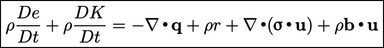(2.51)
The equation describes the rate of change of mechanical and thermal energies for a volume of material of ﬁxed mass of ﬂuid particles. Thermal energy is described by the speciﬁc internal energy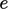, where speciﬁc denotes per unit mass. Mechanical energy is represented by speciﬁc kinetic energy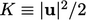.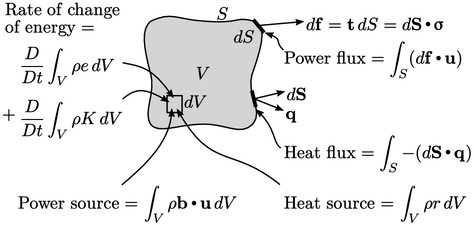The rate of change of these combined energies is due to the input of mechanical power and heat from both surface ﬂuxes and internal sources.

Mechanical power is calculated by the inner product of force and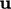. The power over a surface segment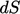is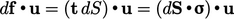, representing a change in strain energy. The internal power source is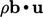, representing a change in potential energy due to general body forces.

Heat is provided by an internal source of strength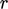per unit mass and a surface heat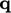per unit surface area. The surface heat ﬂux relating to heat input takes a negative sign,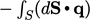, sincefollows the convention of pointing out of the volume.

Equation 2.51 is derived by equating integrals of rate of change of energy to the mechanical power and heat input and taking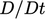inside the volume integral since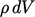is constant in time, as follows: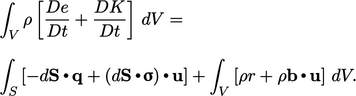(2.52)
Gauss’s theorem converts the surface integrals of Eq. (2.52 ) into the following volume integrals: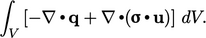(2.53)
Substituting Eq. (2.53 ) for the surface integrals into Eq. (2.52 ) and equating the integrand to 0, as described previously in Sec. 2.7 , yields Eq. (2.51).
13from Hermann von Helmholtz, Über die Erhaltung der Kraft, 1847, who credited it equally to Julius von Mayer, 1842, and James Joule, 1843. “Joule’s apparatus” is the best know demonstration in which the work of a falling weight is converted into heat in water. The ﬁrst law of thermodynamics is the law of conservation of energy applied to a system.

Notes on CFD: General Principles - 2.15 Conservation of energy